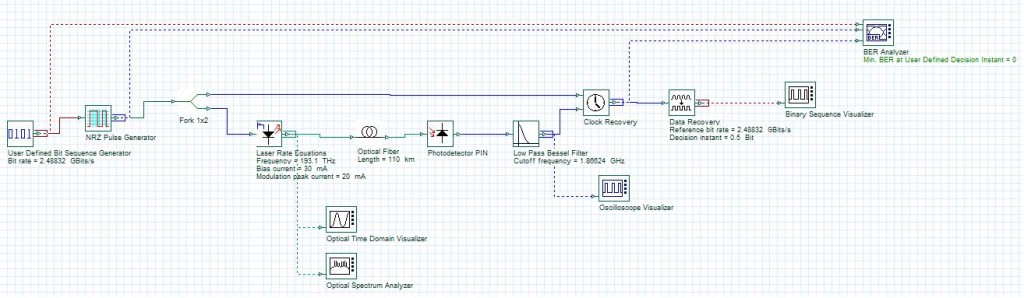BER Analysis Using Matlab®/OptiSystem Automation

This example demonstrates the use of Matlab® software to call OptiSystem and calculate the bit error rate after the OptiSystem simulation.

Samples: MatlabOptiSysBER.m

The Matlab file “MatlabOptiSysBER.m” executes the following functions:

• Generates the data (sequences of bits) that are transmitted through OptiSystem;
• Opens OptiSystem;